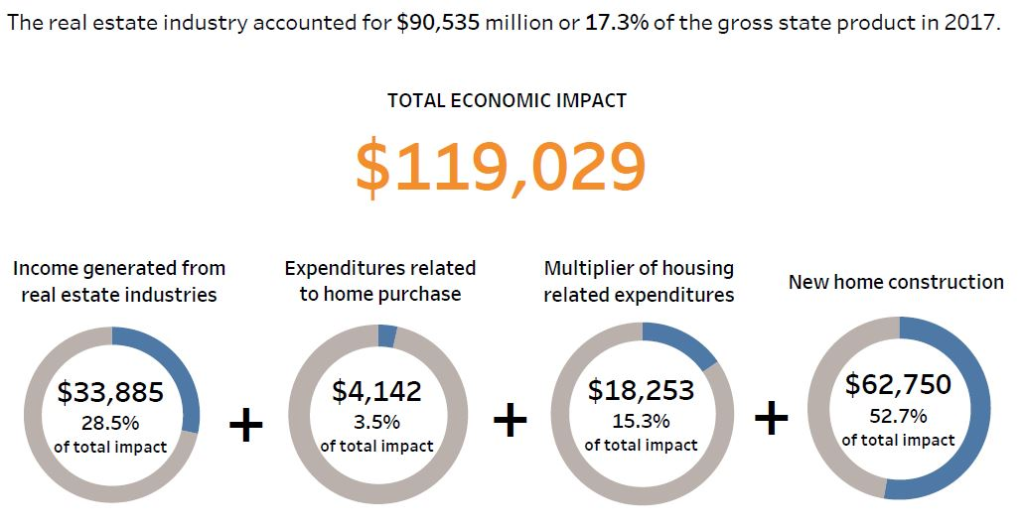# 1. What is the economic multiplier? 2. What is the size multiplier effect? 3. What is the total i...

1. What is the economic multiplier?

2. What is the size multiplier effect?

3. What is the total income derived from a typical home sale?The real estate industry accounted for \$90,535 million or 17.3% of the gross state product in 2017 TOTAL ECONOMIC IMPACT \$119,029 Income generated from real estate industries Multiplier of housing related expenditures Expenditures related New home construction to home purchase \$33,885 28.5% of total impact \$4,142 3.596 of total impact \$18,253 15.3% of total impact \$62,750 52.7% of total impact##### Add Answer of: 1. What is the economic multiplier? 2. What is the size multiplier effect? 3. What is the total i...
More Homework Help Questions Additional questions in this topic.

• #### Explain the difference between the government purchases multiplier and the net tax multiplier

Need Online Homework Help?